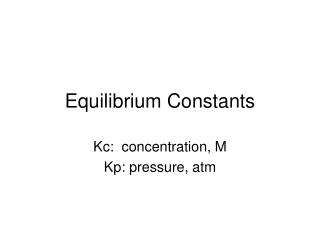DownloadDownload PresentationEquilibrium Constants

# Equilibrium Constants

Télécharger la présentation## Equilibrium Constants

- - - - - - - - - - - - - - - - - - - - - - - - - - - E N D - - - - - - - - - - - - - - - - - - - - - - - - - - -
##### Presentation Transcript

1. Equilibrium Constants Kc: concentration, M Kp: pressure, atm

2. Equilibrium Expressions aA + bB  cC + dD Kc = [C]c[D]dKp = (PC)c(PD)d [A]a[B]b (PA)a(PB)b Kp = Kc(RT)Dn Dn= S gas product coefficients – S gas reactant coefficients *If the substance is a solid or a liquid, put a 1 in its place.

3. Example #1 • Write the equilibrium expressions for Kc for the following. • 2H2O(l) 2H2(g) + O2(g) • 2HCl(aq)  H2(g) + Cl2(g)

4. Example Problem #2 • The following equilibrium concentrations were observed for the Haber process at 127oC N2(g) + 3H2(g) 2NH3 (g) [NH3] = 0.031M [N2] = 0.85M [H2] = 0.0031M Calculate Kc and Kp.

5. Example Problem #3 • The reaction below occurs at 25oC. 2NO(g) + Cl2(g) 2NOCl(g) The equilibrium partial pressures are: PNOCl = 1.2atm PNO = 0.05atm PCl2 = 0.30atm Calculate Kp and Kc.

6. Reaction Quotient • Predicting shifts in equilibrium mathematically Qc = [C]c[D]dQp = (PC)c(PD)d [A]a[B]b (PA)a(PB)b Q<K: shifts right Q>K: shifts left Q=K: at equilibrium

7. Example Problem #4 • The equilibrium constant, Kp, is 2.33 for the reaction C(s) + CO2(g) 2CO(g) at 50oC. If 0.75g of C, 3.5atm of CO2 and 4.5 atm CO are present in a container, is the reaction at equilibrium? If not, which way will it shift?

8. Example #5 The equilibrium constant, Kp, for the reaction 2H2O(l) 2H2(g) + O2(g) is 5 x 10-6. If 50g of hydrogen, 25g of oxygen and 100g of water are placed in a 5L flask at 100oC, will the reaction be at equilibrium? If not, which way will it shift?

9. Example #6 Consider the reaction: N2(g) + 3H2(g) 2NH3 (g) The equilibrium constant, Kc, at 25oC is 0.082. If 0.4mol of nitrogen, 0.098mol of hydrogen and 2.32mol of ammonia are present in a 4L container, is the system at equilibrium? If not, which way will it shift?

10. Determining Equilibrium concentrations • The ICE method aA + bB  cC + dD I # # 0 0 C -ax -bx +cx +dx E #-ax #-bx cx dx I= initial concentrations, C= change during reaction process E = equilibrium concentrations.

11. Example Problem #7 • For the reaction N2O4(g) 2NO2(g) the equilibrium constant, Kp, is 0.133. If 2.71 atm of N2O4 are introduced into a container, what will the equilibrium partial pressure be for all the species in the reaction?

12. Example Problem #8 • For the reaction: CO(g) +H2O(g) CO2(g) + H2 (g) the equilibrium constant, Kc, is 5.10 at 700K. If 3 moles of CO and 3 moles of H2O are introduced into a 5L flask, what will the equilibrium concentrations be for all the species present in the reaction?

13. Example #9 • For the reaction 2NOCl(g) 2NO(g) + Cl2(g) the equilibrium constant, Kc, is 1.6 x 10-5 at 35oC. If 3 moles of NOCl are placed in a 6L container, what will the concentrations of all the species reach at equilibrium?